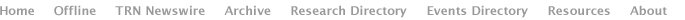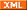## Two schools of cryptography

September 19, 2005
Cryptography is the cornerstone of electronic security. It protects all things digital, from bank records and the information stored in the magnetic stripes on credit cards to computer passwords and Internet connections.

Hard numbers vs. uncertainty

There are two basic cryptography methods: computational and probabilistic.

Computationally secure methods use cryptographic keys that are answers to difficult-to-solve mathematical problems. In order to find the answer to the problem, and thus the key, an eavesdropper would have to sift through a very large number of possibilities. If a problem is difficult enough that it would take a state-of-the-art computer millions of years to sift through all the possibilities, chances are the key is secure. Computationally secure cryptography methods are also referred to as conditional.

The drawback to this type of cryptography is that the difficulty of a problem can lessen as technology advances. A problem that would have taken millions of years to solve a decade ago might no longer provide viable security today.

Probabilistically secure methods use cryptographic keys chosen at random from a fast source of random signals. If a source is fast enough that an eavesdropper cannot record all of it, the probability of the eavesdropper gaining access to a key can be very small even given unlimited time for computations. This type of security is also referred to as information-theoretic, or unconditional, security.

The drawback to probabilistically secure cryptography is that it tends not to be as practical as computationally secure cryptography.

Computing security

Encryption schemes commonly in use today use public-private key encryption schemes, a computationally secure method based on one-way problems.

As the term suggests, such problems are easy to solve in one direction, but extremely difficult in the other. The RSA security algorithm that underlies many of todays schemes uses a mathematical problem similar to factoring. A numbers factors are the numbers that can be multiplied together to get that number. The factors of 15, for instance, are 3 and 5. Given a pair of factors, it is a simple matter to multiply them to get a number. It is much more difficult to find factors for a given number, especially for very large numbers, simply because there are so many possibilities.

Public-private key encryption schemes use the difficult side of a problem - in the case of RSA, a very large number - as a public key, and the easy side of the problem - in the case of RSA, the numbers pair of factors - as the private key.

When Alice wants to send Bob a message, she looks up his public key and uses it to scramble the message. The message can only be unscrambled using the private key, which is very difficult to figure out even though the public key is freely available.

These schemes can be enhanced by having the two parties frequently exchange new public/private keys, and by having the two parties use a shared secret to encrypt their public keys.

Security in probabilities

Probabilistic security relies on public sources of random signals and assumes that all parties have limited storage capacity so that they cannot record all of a sources random signals. Sources of random signals include radio broadcasts of noise and natural radio signals from space.

The scheme works by having two parties tune into a random signal and independently record portions of the signal at randomly selected intervals. The two compare notes to determine which portions they recorded in common and use these to form an encryption key. As long as the signal from the time of the first recording to the time of the last generates more data than an eavesdropper can store, and as long as the two parties share more than a few recordings, the odds against an eavesdropper finding the key are astronomical.

Quantum cryptography

Quantum cryptography works by a similar principle, but with a twist. Instead of storage capacity limiting an eavesdroppers ability to capture the random signal, quantum cryptography relies on the physical impossibility of accurately copying the quantum states of individual photons.

Photons can be polarized in one of two pairs of orientations - horizontal-vertical and the two diagonals - and each pair can be used to represent a 1or a 0. To measure the polarization of a photon, you must choose which pair of orientations to measure, and, because measuring a photon destroys it, if you guess wrong about which pair holds the information you dont get a second chance.

To use a quantum key, a sender transmits a random string of polarized photons and records the orientations. The recipient measures the photons, choosing orientations at random. The sender and recipient compare notes over a public channel, and the sender tells the recipient which orientations he guessed correctly about, and so correctly measured. The correctly measured photons form a string of bits that can be used as an encryption key.

Because measuring a photon destroys the information it contains, an eavesdropper would have to replace the photons she measured, and because she would only be able to correctly measure about half of the photons, she would have to randomly replace the other half. This would mean that an average of 25 percent of the forged photons measured by the recipient would fail to match up with the senders record. If the sender and recipient notice an unusually high error rate when they compare notes, they can assume the key has been compromised and they can discard it and try again.

Boosting privacy

Probabilistic cryptography schemes are theoretically perfect, but in practice, imperfect equipment, noisy communications lines and human error create vulnerabilities, and users have to assume that an eavesdropper could pick up at least some of their communications.

One way to reduce the risk is to use privacy amplification, which distills partially secure shared information into a smaller amount of more highly secured information. Mathematical formulas, called one-way hash functions, turn two or more of the original bits into a single new bit. Even if an eavesdropper knows some of the original bits, she doesnt have enough information to calculate the new bits. The drawback is that this requires sending more bits to generate a key.

Last            Next

News:
Research News Roundup
Research Watch blog

Features:
View from the High Ground Q&A
How It Works

News| Blog| BooksAdvertisements: